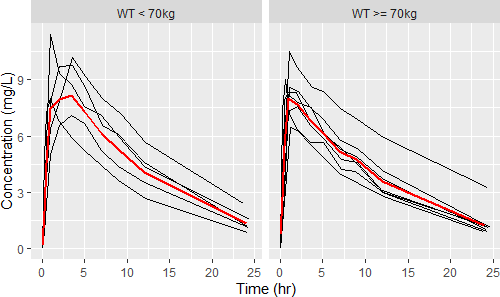## Supp. material + corrections [🇷 for BE/BA]

Hi Weidson,

a couple of problems:
• Typographic quotation marks “ ” – only simple double " " or single quotes ' ' work in any programming language.
• Theoph is no more in package nlme (the ChangeLog doesn’t tell when it was removed). Now it is in package datasets, which is automatically attached.
• You need library(plyr) and a script given in the Supplementary material to create ‘nominal’ times (the ntpd column of the data.frame in the Theoph-list). IMHO, the last cut of 24 is unfortunate, try
   sort(unique(Theoph$ntpd)) with the original code. My quick-and-dirty correction below. • Warning message: fun.y is deprecated. Use fun instead. Such a warning inmeans, that a function or its syntax is still supported but can be removed in the next release of a package without further notice. Hence, never ignore such a warning – always modify the script accordingly. » […] I need of a dinamic code for to plot a graphic of Individual plasma concentration profiles sorted by treatment and period with whih the mean. In practice use the nominal time for grouping. library(ggplot2) # library(nlme) # not needed library(plyr) geomean <- function(x) { if (sum(x <= 0) > 0) x[x <= 0] <- NA return(exp(mean(log(x), na.rm = TRUE))) } Theoph <- datasets::Theoph # only needed if you re-run the code # this is a workaround since the nominal times are unknown Theoph$cut <- cut(Theoph$Time, breaks = c(-0.1, 0, 1, 1.5, 2, 3, 4, 6, 8, 12, 16, 20, 25)) # original 24 is not a good idea tab <- ddply(Theoph, .(cut), summarize, ntpd = round(mean(Time, na.rm = TRUE), 2)) Theoph <- merge(Theoph, tab, by = c("cut"), all.x = TRUE) Theoph <- Theoph[order(Theoph$Subject, Theoph$Time), c("Subject", "ntpd", "Time", "conc", "Dose", "Wt")] Theoph$WT <- ifelse (Theoph$Wt < 70, "WT < 70kg", "WT >= 70kg") p <- ggplot(data = Theoph, aes(x = Time, y = conc, group = Subject)) + geom_line() + labs(x = "Time (hr)", y = "Concentration (mg/L)") + stat_summary(fun = geomean, geom = "line", # original: fun.y = mean aes(x = ntpd, y = conc, group = 1), color = "red", size = 1) p + facet_grid(. ~ WT)However, my workaround is also not ideal. It resolves the issue at the last time point but there are still different concentrations aggregated to the same time point in some subjects, e.g., print(Theoph[Theoph$Subject == 8 & Theoph$Time <= 1, 1:4], row.names = FALSE) Subject ntpd Time conc 8 0.00 0.00 0.00 8 0.52 0.25 3.05 8 0.52 0.52 3.05 8 0.52 0.98 7.31  I couldn’t find the original data set of Robert Upton anywhere. I made an educated guess based on ‘Study D’,1,2 where the weight range of twelve subjects was reported with 55–86 kg. The dose was 320 mg and what is given in Theoph$Dose is actually Dose/kg. After more detective work based on Table I: The product was Slophyllin aqueous syrup (Dooner Laboratories), 80 mg theophylline per 15-ml dose.

Theoph <- datasets::Theoph Theoph <- cbind(Theoph, ntpd = c(0, 0.25, 0.5, 1, 2, 3.5, 5, 7, 9, 12, 24)) # continue with Theoph\$WT <- ...PS: The median in your script is fine. If prefer the geometric mean. Anything is better than the arith­me­tic mean in the original script.PPS: Arghl! Mittyri was faster.1. Upton RA, Sansom L, Guentert TW, Powell JR, Thiercellin J-F, Shah VP, Coates PE, Riegelman S. Evaluation of the Absorption from 15 Commercial Theophylline Products Indicating Deficiencies in Currently Applied Bio­avail­ability Criteria. J Pharmacokin Biopharm. 1980; 8(3): 229–42. doi:10.1007/BF01059644.
2. Interesting: The linear-up / log-down trapezoidal rule was used and $$\small{AUC_{0-\infty}=AUC_{0-\textrm{tlast}}+\frac{\hat{C}_\textrm{tlast}}{k}}$$, i.e., the estimated last concentration in extrapolation. I like that. Furthermore, PK was linear up to 500 mg and hence, additionally $$\small{AUC\times k}$$ was compared (recall this thread).
• […] the assumption of constant clearance in the individual between the occasions of receiving the standard and the test dose is suspect for theophylline. […] If there is evidence that the clearance but not the volume of distribution varies in the individual, the $$\small{AUC\times k}$$ can be used to gain a more precise index of bioavailability than obtainable from $$\small{AUC}$$ alone.

Confirmed, esp. for $$\small{AUC_{0-\infty}}$$:
$$\small{\begin{array}{lc} \textrm{PK metric} & CV_\textrm{geom}\,\%\\\hline AUC_{0-\textrm{tlast}} & {\color{Red} {22.53\%}}\\ AUC_{0-\textrm{tlast}} \times \hat{k} & {\color{Blue} {21.81\%}}\\ AUC_{0-\infty} & {\color{Red} {28.39\%}}\\ AUC_{0-\infty} \times \hat{k} & {\color{Blue} {20.36\%}}\\\hline \end{array}}$$

Dif-tor heh smusma 🖖Helmut SchützThe quality of responses received is directly proportional to the quality of the question asked. 🚮
Science QuotesIng. Helmut Schütz